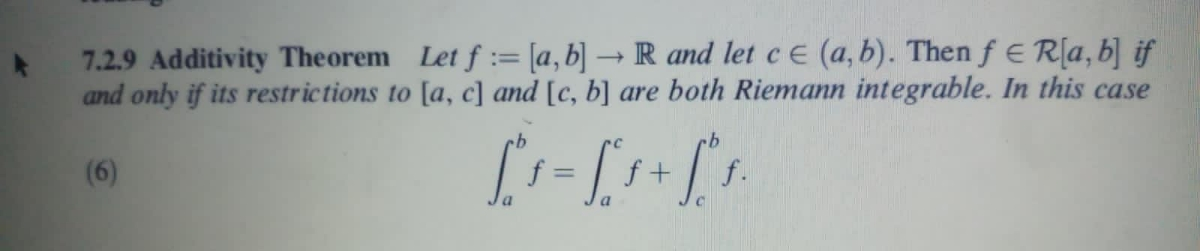# 7.2.9 Additivity Theorem Let f := [a,b] → R and let ce (a, b). Then f E R[a, b] if and only if its restrictions to [a, c] and [c, b] are both Riemann integrable. In this case (6) f + f. %3D

Question
prove this theorem?help_outlineImage Transcriptionclose7.2.9 Additivity Theorem Let f := [a,b] → R and let ce (a, b). Then f E R[a, b] if and only if its restrictions to [a, c] and [c, b] are both Riemann integrable. In this case (6) f + f. %3D fullscreen

1 Rating

### Want to see this answer and more?

Experts are waiting 24/7 to provide step-by-step solutions in as fast as 30 minutes!*

*Response times may vary by subject and question complexity. Median response time is 34 minutes for paid subscribers and may be longer for promotional offers.
Tagged in
Math